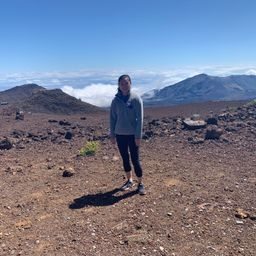# Elizabeth X.

Winsor School

## Biography

Hi! I'm Elizabeth, a senior in high school at the Winsor School. I have experience tutoring other high school students in math as well as chemistry.

BA N/A
Winsor School

## Topics Covered

Vectors
Vector Calculus
The Language of Algebra
Equations and Inequalities
Functions
Rational Functions
Exponential and Logarithmic Functions
Trigonometry
An Introduction to Geometry
Introduction to Trigonometry
Sampling and Data
The Normal Distribution
Introduction to Combinatorics and Probability
Exponents and Polynomials
Limits
Differentiation
Continuous Functions
Graphs and Statistics
Matrices
Systems of Equations and Inequalities
Introduction to Matrices
Parametric Equations
Polar Coordinates
Introduction to Vectors
Complex Numbers